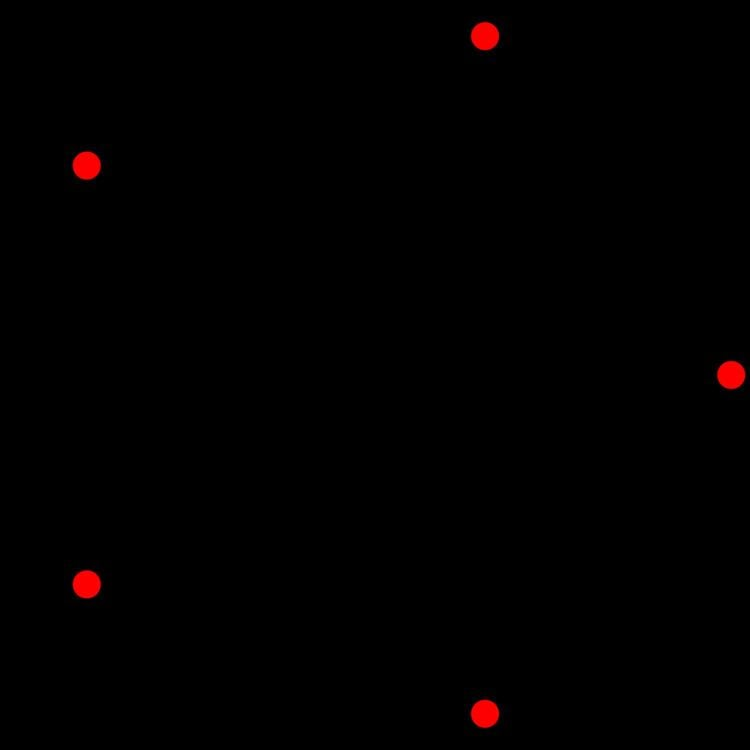# A4 polytope

Updated on
Covid-19In 4-dimensional geometry, there are 9 uniform polytopes with A4 symmetry. There is one self-dual regular form, the 5-cell with 5 vertices.

## Symmetry

A4 symmetry, or [3,3,3] is order 120, with Conway quaternion notation +1/60[I×I].21. Its abstract structure is the symmetric group S5. Three forms with symmetric Coxeter diagrams have extended symmetry, [[3,3,3]] of order 240, and Conway notation ±1/60[I×I].2, and abstract structure S5×C2.

## Visualizations

Each can be visualized as symmetric orthographic projections in Coxeter planes of the A4 Coxeter group, and other subgroups. Three Coxeter plane 2D projections are given, for the A4, A3, A2 Coxeter groups, showing symmetry order 5,4,3, and doubled on even Ak orders to 10,4,6 for symmetric Coxeter diagrams.

The 3D picture are drawn as Schlegel diagram projections, centered on the cell at pos. 3, with a consistent orientation, and the 5 cells at position 0 are shown solid.

## Coordinates

The coordinates of uniform 4-polytopes with pentachoric symmetry can be generated as permutations of simple integers in 5-space, all in hyperplanes with normal vector (1,1,1,1,1). The A4 Coxeter group is palindromic, so repeated polytopes exist in pairs of dual configurations. There are 3 symmetric positions, and 6 pairs making the total 15 permutations of one or more rings. All 15 are listed here in order of binary arithmetic for clarity of the coordinate generation from the rings in each corresponding Coxeter diagram.

The number of vertices can be deduced here from the permutations of the number of coordinates, peaking at 5 factorial for the omnitruncated form with 5 unique coordinate values.

Topics